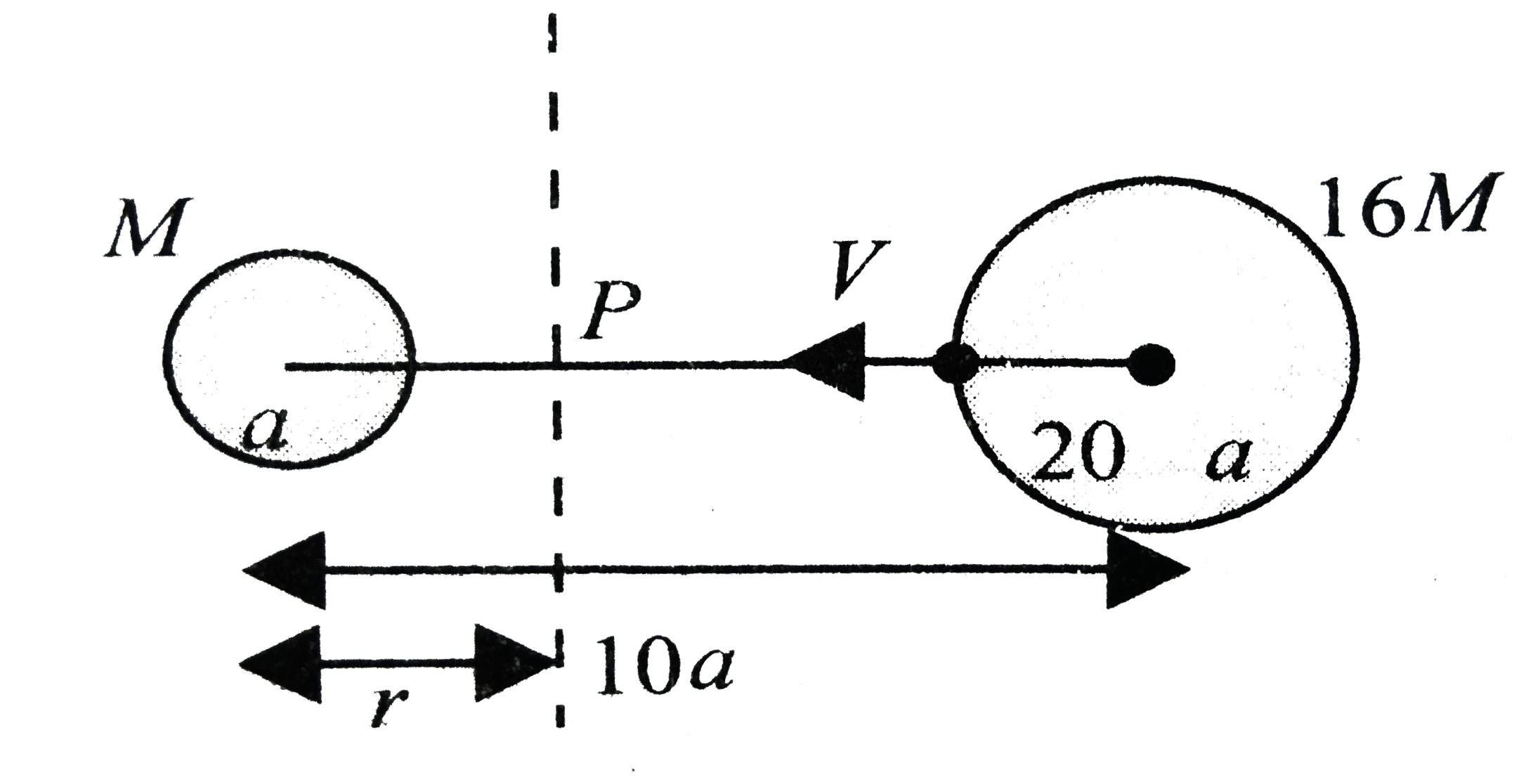# Distance between the centres of two stars is 10a. The masses of these stars are M and 16 M and their radii a and 2a respectively. A body of

46 views
in Physics
closed
Distance between the centres of two stars is 10a. The masses of these stars are M and 16 M and their radii a and 2a respectively. A body of mass m is fired straight from the surface of the larger star towards the surface of the smaller star. What should be its minimum initial speed to reach the surface of the smaller star? Obtain the expression in terms of G, M and a.

by (75.0k points)
selected

Let P be the point on the joining the centres of the two planets such that the net field at that point is zero.Then (GM)/(r^(2))-(G16M)/((10a-r)^(2))=0
implies(10a-r)^(2)=16r^(2)
implies 10a-r=4r
impliesr=2a
Potential at point P
v_(P)=(-GM)/r=(G16M)/((10a-r))=(-GM)/(2a)-(2GM)/a
(-5GM)/(2a)
Now if the particle projected from the larger planet has enough energy to cross this point, it will reach the smaller planet.
For this, the KE imparted to the body must be just enough to raise its total mechanical enrgy to a value which is equal to PE at point P, i.e.
1/2mv^(2)-(G(16M)m)/(2a)-(GMm)/(8a)=mv_(P)
or (v^(2))/2-(8GM)/a-(GM)/(8a)=(5GMm)/(2a)
or v^(2)=(45GM)/(4a)
or v_(min)=3/2sqrt((5GM)/a)